×

Search anything:

# Biconnected Components [Explained + Algorithm to find it]

#### Graph Algorithms AlgorithmsFREE BOOK -> Problems for the day before your Coding Interview (on Amazon)

Turns out, biconnected components are one of the most important topic in design analysis of algorithms, data structures and in many competitive exams.
Biconnected components are defined as a maximal biconnected subgraph.
Lets understand it better!

1. Prerequisites
1.1 Graph
1.2 Articulation Point
1.3 Biconnected Graph
2. What Are Biconnected Components
3. How To Build A Biconnected Graph
4. Identify Articulation Point In A Graph
5. Identify Biconnected Components In A Graph
6. Why These Are Important

# 1 Prerequisites

These are some of the things which you should know before we get to our main topic!

## 1.1 Graph

Graphs can be defined as a mathematical structure which represents a specific function. It is constructed by connecting a set of points using lines. Each points are known as vertex (node) and the line joining it is known as edges. In the below graph A,B,C,D and E are known as vertex and the lines joining them are known as edges.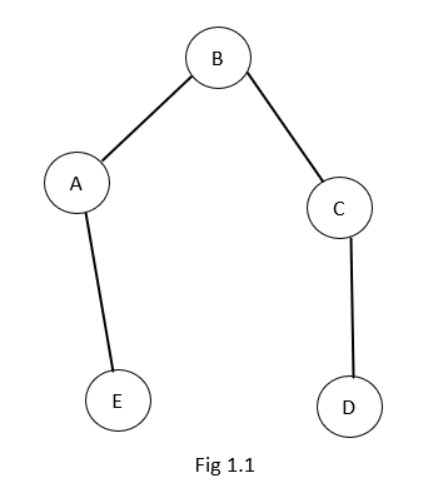These graphs have a lot of applications in a lot of different fields like computer science, physics, chemistry etc. There is even a study called graph theory to learn about the relationship between nodes and edges.

So its a very important concept. The topic biconnected component is a part of this theory. There are a lot of different types of graphs, eg: Conected graph: If in a graph there is a path between every pair of distinct vertices we can call it a connected graph.

## 1.2 Articulation Point

A vertex V in a connected graph G is an articulation point (cut point) if the deletion of vertex V along with all edges incident to V disconnects the graph into two or more non-empty components.

Lets demonstrate it with an example:
Fig 1.2 shows a connected graph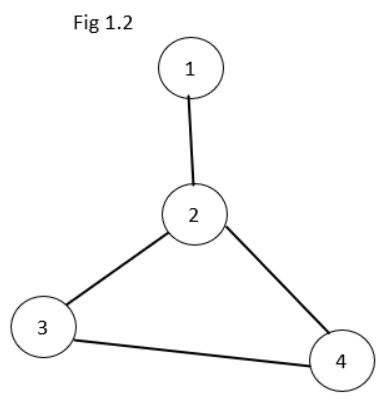Here we can say vertex 2 is an articulation point, because if we remove the vertex 2 along with the incident edges we can observe that the graph splits into two non-empty component.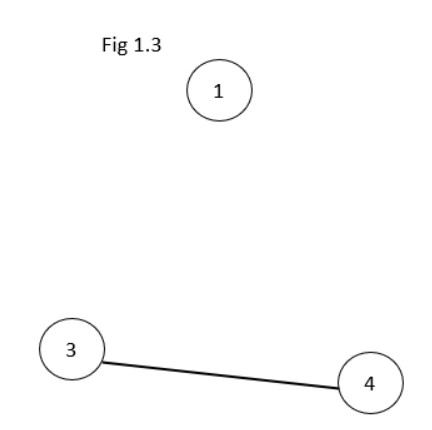After deleting vertex 2 and the incident edges of vertex 2, the given graph is divided into two non-empty components as in the figure above.
=> vertex 2 is an articulation point

## 1.3 Biconnected Graph

We can call a graph G a biconnected graph if it contains zero articulation point.
Example: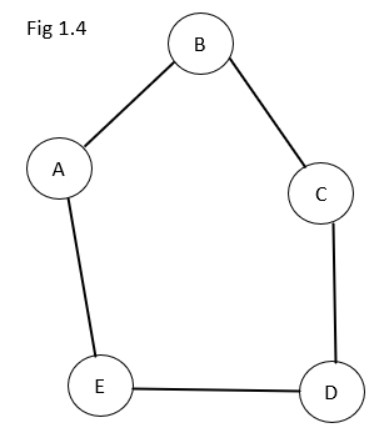The above graph contains no articulation point => The graph is Biconnected Graph.

# 2. What Are Biconnected Components

A maximal biconnected subgraph is a Biconnected component. Its really simple if you understand the sentance carefully.

Take a biconnected sub-graph of a graph, then keep on adding the neighbouring vertices and edges and take a step back if you find an articulation point. So now you will have a biconnected sub-graph of the given graph with maximum nodes possible such that there are no articulation point.

In simple words a biconnected component is a part of a graph that is connected in a special way. Imagine you have a graph with nodes (called vertices) and lines (called edges)
connecting them. A biconnected component is a group of vertices and edges that are all connected to each other in a way that you can always get from one vertex to another using two
different paths.

Note: You might have to read it a couple of times to get the whole idea.
Note From the defenition you might have understood that any biconnected graph can be a biconnected component, as any graph is a sub-graph of itself.

Lets take an example: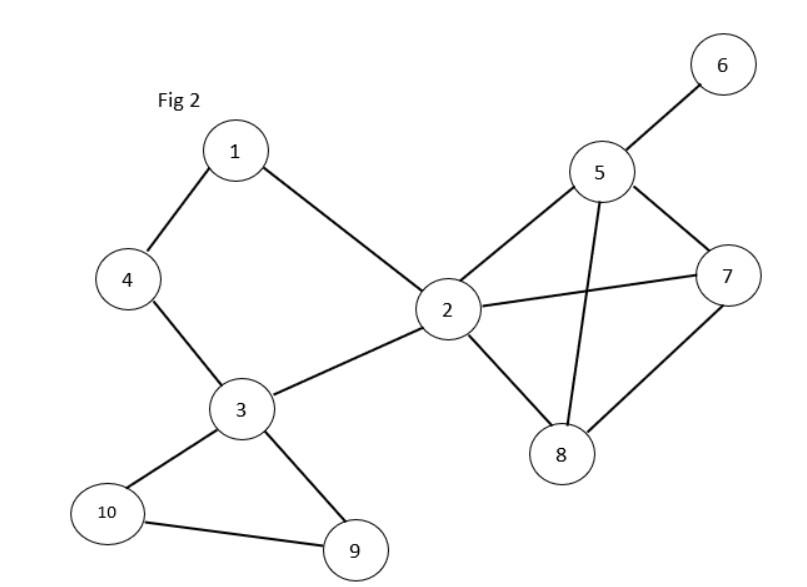Here the given graph has 3 articulation points ie, vertex 2, 3, 5, as the graph splits into various parts if we remove one vertex and the edges incident to it.
So the following are the biconnected components in the graph. As you might observe if we take two of them togeher they will have atmost 1 vertex in common and that vertex is none other than the articulation point. Take any pair and check.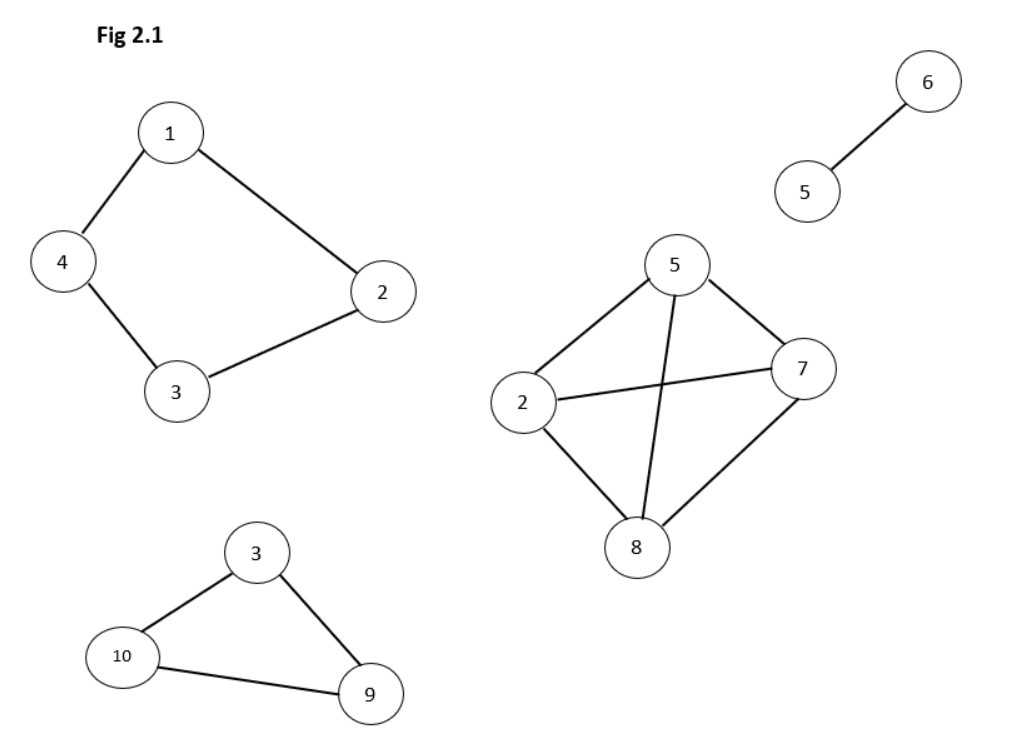# 3. How To Build A Biconnected Graph

Steps to follow to build a biconnected graph:

1. Check whether the given graph is biconnected or not.
2. If its not biconnected identify all the articulation point.
3. After obtaining the articulation points, determine the set of edges whose inclusion makes the graph connected in the absence of articulation point.

Now lets look the steps for our graph (Fig 2).

1. The given grapg G is not a biconnected graph, the articulation points are 2,3 and 5.
2. To transform G to biconnected graph new edges are included to te articulation point.
• Edges corresponding to the articulation point 2 are (1,5) and (3,8).
• Edges corresponding to the articulation point 3 are (4,10) and (2,9).
• Edges corresponding to the articulation point 5 are (6,7).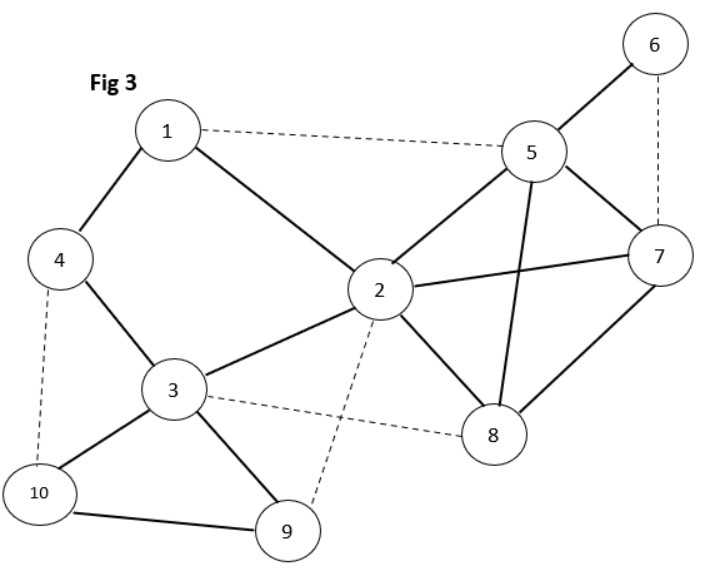The edges added are represented as dotted lines.
After the edition of these lines we can observe that it is a biconnected graph.

# 4. Identify Articulation Point In A Graph

An articulation point (or cut vertex) in a graph is a vertex that, when removed, increases the number of connected components in the graph. In other words, an articulation point is a vertex that "separates" the graph into two or more separate parts, and if it is removed, those parts will become disconnected.

One of the simplest algorithms for identifying articulation points in a given graph is to perform a depth-first search (DFS) traversal of the graph and keep track of the following information for each vertex:

1. The DFS number of the vertex: This is the order in which the vertex was visited during the DFS traversal.
2. The low-link value of the vertex: This is the minimum DFS number of any vertex that can be reached from the current vertex by following zero or more DFS tree edges and then at least one back edge.

To identify the articulation points in the graph, we can use the following steps:

1. Start by setting the DFS number and low-link value of each vertex to be the same.
2. For each vertex, do the following:
a. Consider all of the vertices that are reachable from the current vertex by a single edge.
b. For each of these vertices, compute the low-link value using the following rule:
• If the low-link value of the current vertex is greater than the low-link value of the reachable vertex, set the low-link value of the current vertex to be the same as the low-link value of the reachable vertex.
• If the low-link value of the current vertex is equal to its DFS number, then it is an articulation point.
3. Repeat this process for all vertices in the graph.

This algorithm has a time complexity of O(V+E), where V is the number of vertices and E is the number of edges in the graph. This is because the algorithm performs a depth-first search (DFS) traversal of the graph, which has a time complexity of O(V+E). The space complexity of the algorithm is also O(V+E), as it stores the DFS number and low-link value for each vertex in the graph.

The algorithm described above will work for any type of graph, including directed and undirected graphs, as well as graphs with self-loops and multiple edges.

# 5. Identify Biconnected Components

Let's look at how to find a biconnected component in a graph. For this we will use Tarjan's algorithm for finding biconnected components in a graph. It was developed by Robert Tarjan in 1972.

Algorithmic steps:

1. Initialize a counter `dfn` to 1, which will be used to assign a depth-first search (DFS) number to each vertex. Initialize an empty stack `S` and a list of biconnected components `components`.

2. Run a DFS on the graph, keeping track of the following information for each vertex `v`:

• `dfn[v]`: the DFS number of `v`
• `low[v]`: the lowest DFS number reachable from `v`
• `parent[v]`: the parent of `v` in the DFS tree
3. For each vertex `v` visited in the DFS, do the following:

• If `v` is the root of the DFS tree, and it has more than one child, add all of its children to the current biconnected component `C`.
• If `v` is not the root of the DFS tree, and `low[v] >= dfn[v]`, then `v` is an articulation point. In this case, add `v` and all vertices on the stack `S` that have a DFS number greater than `low[v]` to the current biconnected component `C`.
4. For each edge `(u, v)` in the graph, do the following:

• If `dfn[u] < low[v]`, then `(u, v)` is a back edge. In this case, set `low[v] = dfn[u]`.
• If `dfn[u] > dfn[v]`, then `(u, v)` is a forward edge. In this case, push `v` onto the stack `S`.
5. When the DFS is complete, the list of biconnected components components will contain all of the biconnected `components` of the graph.

Lets look at the C++ imlementation of this:

``````#include <iostream>
#include <vector>
#include <stack>

using namespace std;

const int N = 1e5 + 5;

int n, m;
int dfn[N], low[N], parent[N], timeStamp;
stack<int> S;
vector<vector<int>> components;

void dfs(int u) {
dfn[u] = low[u] = ++timeStamp;
S.push(u);

for (int v : adj[u]) {
if (dfn[v] == 0) {
parent[v] = u;
dfs(v);
low[u] = min(low[u], low[v]);
if (low[v] >= dfn[u]) {
vector<int> component;
int w;
do {
w = S.top();
S.pop();
component.push_back(w);
} while (w != v);
component.push_back(u);
components.push_back(component);
}
} else if (v != parent[u]) {
low[u] = min(low[u], dfn[v]);
}
}
}

int main() {
cin >> n >> m;
for (int i = 0; i < m; i++) {
int u, v;
cin >> u >> v;
}

for (int i = 1; i <= n; i++) {
if (dfn[i] == 0) {
dfs(i);
}
}

for (const auto& component : components) {
for (int v : component) {
cout << v << " ";
}
cout << endl;
}

return 0;
}
``````

This code first reads in the number of vertices `n` and edges `m` in the graph, and then reads in the edges of the graph. It then runs a DFS on the graph and uses the stack `S` and the arrays `dfn`, `low`, and `parent` to identify the biconnected components of the graph. Finally, it prints out the vertices in each biconnected component.

Here is an example of input and output for this code:

Input:

``````6 7
1 2
2 3
3 1
3 4
4 5
5 6
6 4
``````

Output:

``````1 3 2
4 6 5
``````

This input represents a graph with 6 vertices and 7 edges, with biconnected components consisting of the vertices 1, 2, 3 and 4, 5, 6. The output correctly lists these biconnected components.

The time complexity of Tarjan's algorithm is O(V + E), where V is the number of vertices and E is the number of edges in the graph. The space complexity is also O(V + E), as the algorithm uses an adjacency list to represent the graph and a stack to store the vertices on the current DFS path.

# 6. Why These Are Important?

There are many applications of these in many fields.

An articulation point (or cut vertex) in a graph is a vertex that, when removed along with all the edges incident to it, increases the number of connected components in the graph. In other words, an articulation point is a vertex that plays a key role in keeping the graph connected.

Biconnected components, on the other hand, are subgraphs of a graph that are themselves connected and do not have any articulation points.

These concepts are important because they can be used to analyze the connectivity and structural properties of a graph. For example, an articulation point can be used to identify the most critical vertices in a network, such as bridges in a road network or routers in a computer network. Biconnected components can be used to identify the most robust parts of a graph, as they remain connected even if one of their vertices is removed.

In addition, algorithms for finding articulation points and biconnected components are important building blocks for other graph algorithms, such as finding bridges and cycles in a graph, and for analyzing the structure of graphs in general.

By the end of this article at OpenGenus, you must have complete knowledge on biconnected components, lets test your knowledge with a question!

## Question

#### You are given a biconnected graph G. How many biconnected components are there in it?

1
2
v-1 where v is the number of vertices
Square root of v where v is the number of vertices
1 is the correct answer. Since it is a biconnected graph, the graph itself would be the only biconnected component possible.

#### Aswin Shailajan

Aswin Shailajan is a B. Tech Computer Science student at Vellore Institute of Technology and is a SDE, Intern at OpenGenus.

Biconnected Components [Explained + Algorithm to find it]